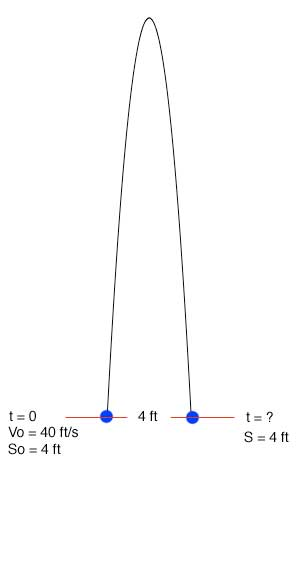SEARCH HOMEMath Central Quandaries & QueriesDavid, a student: A juggler tosses a ball into the air with a velocity of 40ft/sec from a height of 4 ft. Use s= -6t2 + vot+so to find how long it takes for the ball to return to the height of 4 ft. I was wondering how should i start this out, and can you give me a brief explanation on the formula they ask you to use? thxHi David,

You are actually given the formula to find time: s= -6t2 + vot+so

 s - distance at time t. In this case we want to find t when s = 4 ft vo - initial velocity is given to be 40 ft/s so - initial distance is given to be 4 ft Now it is just a matter of plugging the given information into the formula: 4= -6t2 + 40t+4 Now you can collect like terms and solve by factoring or using the quadratic equation.Hope this helps,

JaniceMath Central is supported by the University of Regina and The Pacific Institute for the Mathematical Sciences.